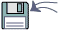Model Explorer for Econometric Views 3.

 Model Explorer will generate a custom program written in the Econometric Views language. This program will allow you to estimate EVERY POSSIBLE regression model mixing a given group of variables. Running millions of test regressions is easy.Model Explorer for Econometric Views 3. download will automatically start in 5 sec... Click here if it does not
 Model Explorer for Econometric Views Related Software [web:reg] arma add-in 1.0The parameter of an pure AR(p) model can be estimated by OLSDataFit 9.0DataFit is a tool used to perform nonlinear regression (curve fitting), statistical analysis and data plottingLinear Regression 1.00Performs linear regression using the Least Squares methodAnalysis Studio 6.30.3546.Analysis Studio provides the researcher a fast reliable way to create and manage data mining projects and includes automated methods for gaining a high level of accuracy and model stabilityMultiple Regression Analysis and Forecasting 1. The Multiple Regression Analysis and Forecasting model provides a solid basis for identifying value drivers and forecasting business plan dataRegression Analysis and Forecasting 1.0The Multiple Regression Analysis and Forecasting model provides a solid basis for identifying value drivers and forecasting business plan dataRegression Analysis and Forecasting 2.0The Multiple Regression Analysis and Forecasting model provides a solid basis for identifying value drivers and forecasting business plan dataSTATOOL Statistics and Probability Tools 1.7StaTool - Statistics and Probability Tools for Windows- Hypothesis testing - Confidence interval estimation - Probability distributions - One variable statistics - Two variables statistics - Total Probability Law and Bayes' TheoremStatistic and Probability Tools 1.7StaTool - Statistic and Probability Tools for Windows- Hypothesis testingRegression analysis - CurveFitter 4.5.6CurveFitter program performs statistical regression analysis to estimate the values of parameters for linear, multivariate, polynomial, exponential and nonlinear functions